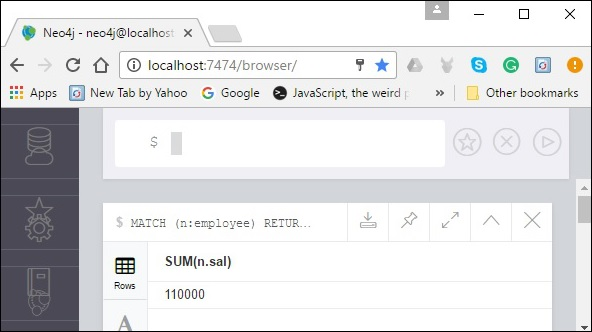# Neo4j - Sum Function

It takes a set of rows and a <property-name> of a node or relationship as an input and finds the summation value from the given <property-name> column of given rows.

## Syntax

Following is the syntax of the SUM() function.

```SUM(<property-name>)
```

## Example

Following is a sample Cypher query which demonstrates the usage of the function SUM() in Neo4j. Here we are trying to calculate the SUM of the salaries of the employees

```MATCH (n:employee) RETURN SUM(n.sal)
```

To execute the above query, carry out the following steps −

Step 1 − Open the Neo4j desktop App and start the Neo4j Server. Open the built-in browser app of Neo4j using the URL http://localhost:7474/ as shown in the following screenshot.Step 2 − Copy and paste the desired query in the dollar prompt and press the play button (to execute the query) highlighted in the following screenshot.## Result

On executing, you will get the following result.neo4j_aggregation_function.htm